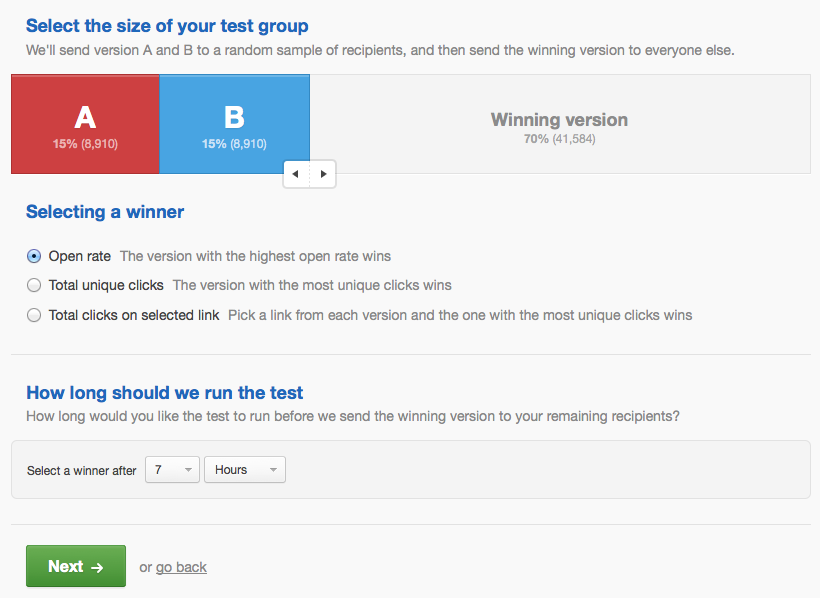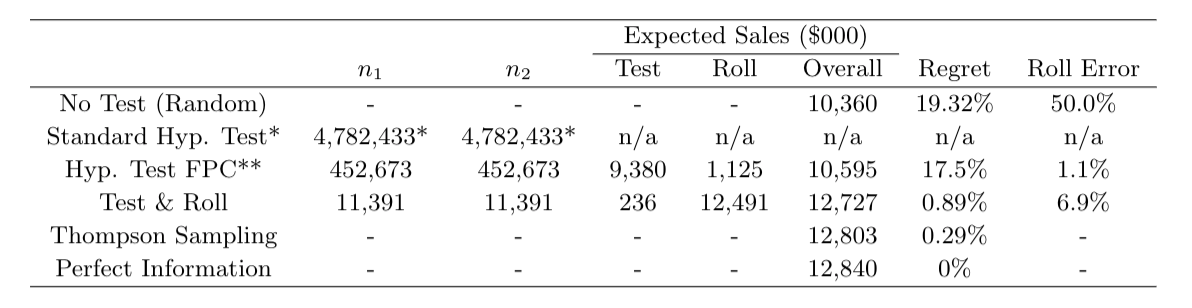6/16/2019

Typical A/B email test setup screenHypothesis testing doesn’t quite fit this problem

1. Hypothesis tests focus on minimizing Type I error
• Doesn’t matter when we are deciding which of two equal-cost treatments to deploy
2. Populations are limited and hypothesis tests don’t recognize this
• Sample size formulas will suggest sample sizes larger than the population
3. When a hypothesis test is insignificant, it doesn’t tell you what to do.
• Choose randomly? That doesn’t make sense!
4. Doesn’t allow for unequal group sizes
• But we see these all the time in media holdout testing

A/B tests as a decision problem

Test

Choose $$n_1^*$$ and $$n_2^*$$ customers to send the treatments.
Collect data on response.

Roll

Choose a treatment to deploy to the remaining $$N - n_1^* - n_2^*$$.

Objective

Maximize combined profit for test stage and the roll stage.

Profit-maximizing sample size

For the case where response is normally distributed with variance $$s$$ and a symmetric normal prior on the mean response ($$m_1, m_2 \sim N(\mu, \sigma)$$), the profit maximizing sample size is

$n_1 = n_2 = \sqrt{\frac{N}{4}\left( \frac{s}{\sigma} \right)^2 + \left( \frac{3}{4} \left( \frac{s}{\sigma} \right)^2 \right)^2 } - \frac{3}{4} \left(\frac{s}{\sigma} \right)^2$ If the priors are different for each group (eg a holdout test), the optimal sample sizes can be found numerically. This new sample size formula was recently derived by Feit and Berman (2019) Marketing Science.

Test & Roll in math

Response
$y_1 \sim N(m_1, s) \,\,\,\,\,\,\, y_2 \sim N(m_2, s)$

Priors
$m_1 \sim N(\mu, \sigma) \,\,\,\,\,\,\, m_2 \sim N(\mu, \sigma)$

Profit-maximizing sample size $n_1 = n_2 = \sqrt{\frac{N}{4}\left( \frac{s}{\sigma} \right)^2 + \left( \frac{3}{4} \left( \frac{s}{\sigma} \right)^2 \right)^2 } - \frac{3}{4} \left(\frac{s}{\sigma} \right)^2$

Interpreting the sample size formula

Bigger population ($$N$$) $$\rightarrow$$ bigger test

More noise in the repsonse ($$s$$) $$\rightarrow$$ bigger test

More prior difference between treatments ($$\sigma$$) $$\rightarrow$$ smaller test

$n_1 = n_2 = \sqrt{\frac{N}{4}\left( \frac{s}{\sigma} \right)^2 + \left( \frac{3}{4} \left( \frac{s}{\sigma} \right)^2 \right)^2 } - \frac{3}{4} \left(\frac{s}{\sigma} \right)^2$

Test & Roll procedure

1. Come up with priors distributions for each treatment
• Use past data, if you’ve got it
2. Use the priors to compute the optimal sample size
3. Run the test
4. Deploy the treatment with the higher posterior to the remainder of the population
• Priors are symmetric $$\rightarrow$$ pick the treatment with the higher average

Come up with priors

Hierarchical Stan model for past experiments

// Stan code for Lewis and Rao 2015 data
// L&R only report the mean and standard deviation for the control group for each experiment
data {
int<lower=1> nexpt; // number of experiments
real<lower=2> nobs[nexpt]; // sample size for control group
real ybar[nexpt]; // observed mean for control group
real<lower=0> s[nexpt]; // observed standard deviation for experiment (pooled)
}
parameters {
real m[nexpt]; // true mean for control group in experiment
real mu; // mean across experiments
real<lower=0> sigma; //standard deviation across experiments
}
model {
// priors
mu ~ normal(0, 10);
sigma ~ normal(0, 3);
// likelihood
for (i in 1:nexpt) {
m[i] ~ normal(mu, sigma);
ybar[i] ~ normal(m[i], s[i]/sqrt(nobs[i]));
}
}

Fit hierarchical model to past experiments

# data taken from tables 1 and 2 of Lewis and Rao (2015)
c <- c(1:3,5:6) # include only advertiser 1 and eliminate exp 4
d1 <- list(nexpt=length(c), nobs=lr$n1[c], ybar=lr$m[c], s=lr$s[c]) m1 <- stan(file="test_roll_model.stan", data=d1, seed=20030601, iter=10000) ## ## SAMPLING FOR MODEL 'test_roll_model' NOW (CHAIN 1). ## Chain 1: ## Chain 1: Gradient evaluation took 2.5e-05 seconds ## Chain 1: 1000 transitions using 10 leapfrog steps per transition would take 0.25 seconds. ## Chain 1: Adjust your expectations accordingly! ## Chain 1: ## Chain 1: ## Chain 1: Iteration: 1 / 10000 [ 0%] (Warmup) ## Chain 1: Iteration: 1000 / 10000 [ 10%] (Warmup) ## Chain 1: Iteration: 2000 / 10000 [ 20%] (Warmup) ## Chain 1: Iteration: 3000 / 10000 [ 30%] (Warmup) ## Chain 1: Iteration: 4000 / 10000 [ 40%] (Warmup) ## Chain 1: Iteration: 5000 / 10000 [ 50%] (Warmup) ## Chain 1: Iteration: 5001 / 10000 [ 50%] (Sampling) ## Chain 1: Iteration: 6000 / 10000 [ 60%] (Sampling) ## Chain 1: Iteration: 7000 / 10000 [ 70%] (Sampling) ## Chain 1: Iteration: 8000 / 10000 [ 80%] (Sampling) ## Chain 1: Iteration: 9000 / 10000 [ 90%] (Sampling) ## Chain 1: Iteration: 10000 / 10000 [100%] (Sampling) ## Chain 1: ## Chain 1: Elapsed Time: 0.215839 seconds (Warm-up) ## Chain 1: 0.221814 seconds (Sampling) ## Chain 1: 0.437653 seconds (Total) ## Chain 1: ## ## SAMPLING FOR MODEL 'test_roll_model' NOW (CHAIN 2). ## Chain 2: ## Chain 2: Gradient evaluation took 8e-06 seconds ## Chain 2: 1000 transitions using 10 leapfrog steps per transition would take 0.08 seconds. ## Chain 2: Adjust your expectations accordingly! ## Chain 2: ## Chain 2: ## Chain 2: Iteration: 1 / 10000 [ 0%] (Warmup) ## Chain 2: Iteration: 1000 / 10000 [ 10%] (Warmup) ## Chain 2: Iteration: 2000 / 10000 [ 20%] (Warmup) ## Chain 2: Iteration: 3000 / 10000 [ 30%] (Warmup) ## Chain 2: Iteration: 4000 / 10000 [ 40%] (Warmup) ## Chain 2: Iteration: 5000 / 10000 [ 50%] (Warmup) ## Chain 2: Iteration: 5001 / 10000 [ 50%] (Sampling) ## Chain 2: Iteration: 6000 / 10000 [ 60%] (Sampling) ## Chain 2: Iteration: 7000 / 10000 [ 70%] (Sampling) ## Chain 2: Iteration: 8000 / 10000 [ 80%] (Sampling) ## Chain 2: Iteration: 9000 / 10000 [ 90%] (Sampling) ## Chain 2: Iteration: 10000 / 10000 [100%] (Sampling) ## Chain 2: ## Chain 2: Elapsed Time: 0.212856 seconds (Warm-up) ## Chain 2: 0.239993 seconds (Sampling) ## Chain 2: 0.452849 seconds (Total) ## Chain 2: ## ## SAMPLING FOR MODEL 'test_roll_model' NOW (CHAIN 3). ## Chain 3: ## Chain 3: Gradient evaluation took 9e-06 seconds ## Chain 3: 1000 transitions using 10 leapfrog steps per transition would take 0.09 seconds. ## Chain 3: Adjust your expectations accordingly! ## Chain 3: ## Chain 3: ## Chain 3: Iteration: 1 / 10000 [ 0%] (Warmup) ## Chain 3: Iteration: 1000 / 10000 [ 10%] (Warmup) ## Chain 3: Iteration: 2000 / 10000 [ 20%] (Warmup) ## Chain 3: Iteration: 3000 / 10000 [ 30%] (Warmup) ## Chain 3: Iteration: 4000 / 10000 [ 40%] (Warmup) ## Chain 3: Iteration: 5000 / 10000 [ 50%] (Warmup) ## Chain 3: Iteration: 5001 / 10000 [ 50%] (Sampling) ## Chain 3: Iteration: 6000 / 10000 [ 60%] (Sampling) ## Chain 3: Iteration: 7000 / 10000 [ 70%] (Sampling) ## Chain 3: Iteration: 8000 / 10000 [ 80%] (Sampling) ## Chain 3: Iteration: 9000 / 10000 [ 90%] (Sampling) ## Chain 3: Iteration: 10000 / 10000 [100%] (Sampling) ## Chain 3: ## Chain 3: Elapsed Time: 0.18011 seconds (Warm-up) ## Chain 3: 0.188699 seconds (Sampling) ## Chain 3: 0.368809 seconds (Total) ## Chain 3: ## ## SAMPLING FOR MODEL 'test_roll_model' NOW (CHAIN 4). ## Chain 4: ## Chain 4: Gradient evaluation took 9e-06 seconds ## Chain 4: 1000 transitions using 10 leapfrog steps per transition would take 0.09 seconds. ## Chain 4: Adjust your expectations accordingly! ## Chain 4: ## Chain 4: ## Chain 4: Iteration: 1 / 10000 [ 0%] (Warmup) ## Chain 4: Iteration: 1000 / 10000 [ 10%] (Warmup) ## Chain 4: Iteration: 2000 / 10000 [ 20%] (Warmup) ## Chain 4: Iteration: 3000 / 10000 [ 30%] (Warmup) ## Chain 4: Iteration: 4000 / 10000 [ 40%] (Warmup) ## Chain 4: Iteration: 5000 / 10000 [ 50%] (Warmup) ## Chain 4: Iteration: 5001 / 10000 [ 50%] (Sampling) ## Chain 4: Iteration: 6000 / 10000 [ 60%] (Sampling) ## Chain 4: Iteration: 7000 / 10000 [ 70%] (Sampling) ## Chain 4: Iteration: 8000 / 10000 [ 80%] (Sampling) ## Chain 4: Iteration: 9000 / 10000 [ 90%] (Sampling) ## Chain 4: Iteration: 10000 / 10000 [100%] (Sampling) ## Chain 4: ## Chain 4: Elapsed Time: 0.231227 seconds (Warm-up) ## Chain 4: 0.242515 seconds (Sampling) ## Chain 4: 0.473742 seconds (Total) ## Chain 4: Fitted model summary(m1)$summary[,c(1,3,5,8)]
##             mean         sd        25%      97.5%
## m    9.490377 0.08467993   9.433258   9.655464
## m   10.500796 0.10008523  10.433861  10.698501
## m    4.860131 0.06181156   4.818066   4.981305
## m   11.470157 0.07017636  11.422815  11.609507
## m   17.615434 0.09047702  17.553716  17.792088
## mu     10.352358 2.00230021   9.138484  14.169450
## sigma   4.398852 1.17817514   3.540116   7.193112
## lp__  -13.182626 1.90558956 -14.218789 -10.511587

Compute optimal sample size

source("nn_functions.R")
(n <- test_size_nn(N=1000000, s=mean(d1$s), mu=10.36044, sigma=4.39646)) ##  11390.89 11390.89 Evaluate the test (eval <- test_eval_nn(n=n, N=1000000, s=mean(d1$s), mu=10.36044, sigma=4.3964))
##         n1       n2 profit_per_cust   profit profit_test profit_deploy
## 1 11390.89 11390.89        12.72715 12727151    236029.3      12491122
##   profit_rand profit_perfect profit_gain      regret error_rate deploy_1_rate
## 1    10360440       12840843   0.9541639 0.008853939 0.06927924           0.5
##   tie_rate
## 1        0

Compare to sample size for hypothesis test

Null hypothesis test size to detect difference between:
- display ads that have no effect - display ads that are exactly worth the costs (ROI = 0 versus ROI = -100).

margin <- 0.5
d <- mean(lr$cost[c])/margin (n_nht <- test_size_nht(s=mean(d1$s), d=d))
##  4782433

Sample size for hypothesis test with finite population correction

(n_fpc <- test_size_nht(s=mean(d1$s), d=d, N=1000000)) ##  452673.4 (eval_fpc <- test_eval_nn(c(n_fpc, n_fpc), N=1000000, s=mean(d1$s), mu=10.36044, sigma=4.39646))
##         n1       n2 profit_per_cust   profit profit_test profit_deploy
## 1 452673.4 452673.4        10.59508 10595077     9379790       1215287
##   profit_rand profit_perfect profit_gain    regret error_rate deploy_1_rate
## 1    10360440       12840877  0.09459509 0.1748946 0.01116195           0.5
##   tie_rate
## 1        0